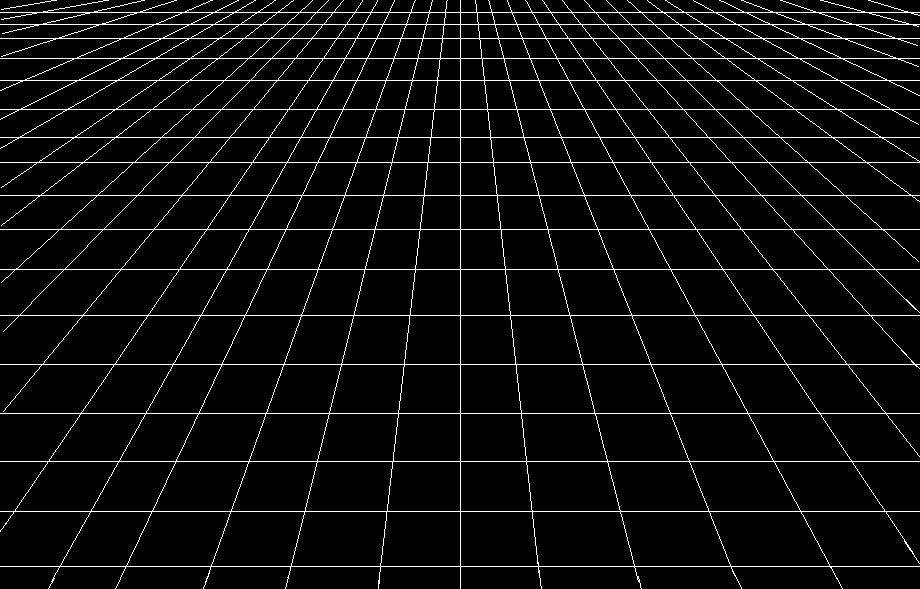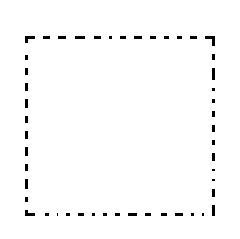# Flat Stanley

In Cosmology by Brian Koberlein4 Comments

One of the things we often say about the universe is that it is “flat.” Of course this usually raises the question about what that actually means.  How can space be flat? Sure, a surface such as a table can be flat, but space?It turns out that the two are somewhat related. If you look at the surface of a table, it’s pretty easy to see that it is flat, at least in a basic sense.  But what if you were a flat creature living on the table’s surface? How would you determine that it is flat? One thing you could do is to mark a starting point, then go forward for a set distance, turn 90 degrees, go the same distance, turn another 90 degrees, same distance again, a final 90 degrees, and then the same distance. What you would find is that you’ve traced out a square to arrive at your starting point.

Suppose you did the same thing on the Earth (treating it like a sphere for a moment). You could walk along the equator for a quarter of the Earth’s circumference, turn 90 degrees and walk north until you reach the north pole, then turn 90 degrees and walk straight to return to the equator.  Again, you return to your starting point, but in this case it only took two turns, not three. That’s because the surface of the Earth is not flat.

More generally, we can do a similar thing with triangles.  Any three points on a flat surface can be connected to form a triangle, and the angles of a flat triangle will always add to 180 degrees. If a surface is curved, such as the surface of the Earth, then the angles will add to more than (positively curved) or less than (negatively curved) 180 degrees.  What makes this useful is that it isn’t limited to surfaces.  Any three points in space can be connected to form a triangle, and if space is “flat” then the angles will add to 180 degrees. In principle we could use three laser-bearing spacecraft to determine the local flatness of space.We could use lasers to determine flat space. Credit: NASA

Given all that, how do we know the universe as a whole is flat? One way is to look at the distribution of galaxies in the universe.  One principle of cosmology is that on large enough scales the universe is homogeneous and isotropic. In other words, galaxies are distributed fairly evenly, and the universe looks pretty much the same in all directions.  That isn’t true on the scale of galaxy clusters, but on a cosmic scale across billions of light years it seems to be true.  If the universe wasn’t flat, then the light from distant galaxies would be distorted similar to gravitational lensing, but on a cosmic scale. We don’t see such an effect, so it seems the universe is flat. A more precise test of cosmic flatness uses the cosmic microwave background (CMB). From fluctuations in the CMB we can determine both the Hubble constant of the universe and the mass density. From these we can calculate the flatness of the universe. What we find is that to the limits of observation, the universe appears to be flat.

Although we know with high certainty that the universe is flat (or very, very close to flat), we still aren’t sure why it’s flat. It is sometimes referred to as the flatness problem, and it remains an unsolved mystery in cosmology.

1.Hi! Recently found out about your blog, it is extraordinary. I have a question regarding this flatness of space – does it somehow relate to curvature of space and time caused by matter? If it is, would it be possible e.g. to measure gravity by measuring angles in triangle in space? (this might be a bit silly question, but I had to ask)

1.Author

Thanks, I’m glad you like it. Using triangles to measure gravity is an idea that may be implemented. The LISA project plans to use three satellites forming a triangle to detect gravitational waves.

1.Very interesting. Thanks for the reply.

2.Hi. But the Hubble constant derived from CMB type measurements are significantly different from optical wavelength measurements. We know that plasmas can change the phase and group velocity of electromagnetic waves. A sub-CMB (<< 1 GHz) may show a different result. I propose a CMRF experiment at about 1-10 MHz.